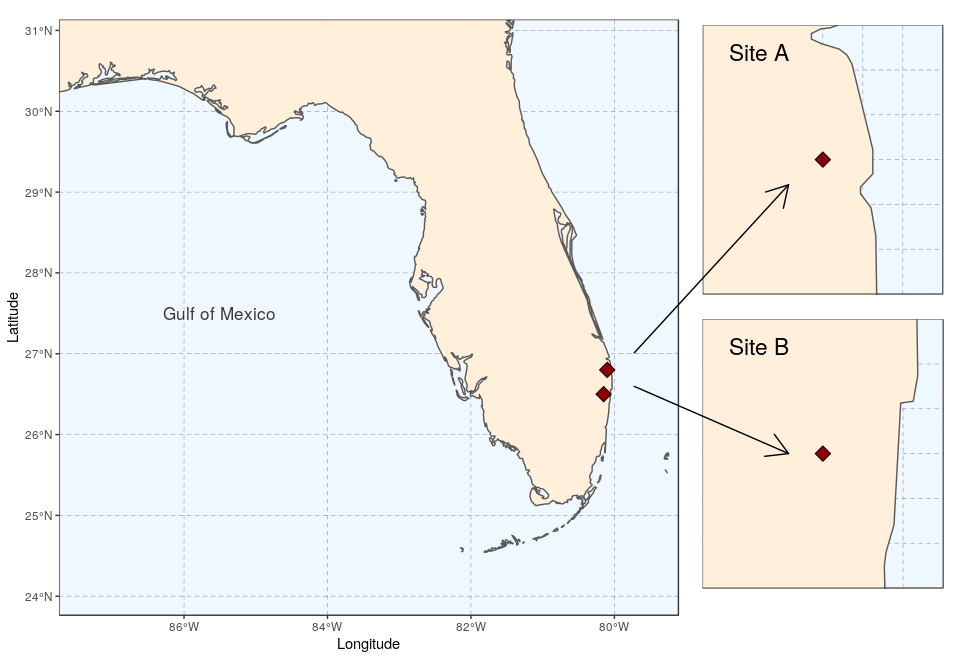Want to share your content on R-bloggers? click here if you have a blog, or here if you don't.

view raw Rmd

This tutorial is the third part in a series of three:

After the presentation of the basic map concepts, and the flexible
approach in layer implemented in `ggplot2`, this part illustrates how to
achieve complex layouts, for instance with map insets, or several maps
combined. Depending on the visual information that needs to be
displayed, maps and their corresponding data might need to be arranged
to create easy to read graphical representations. This tutorial will
provide different approaches to arranges maps in the plot, in order to
make the information portrayed more aesthetically appealing, and most
importantly, convey the information better.

## Getting started

Many R packages are available from CRAN,
the Comprehensive R Archive Network, which is the primary repository of
R packages. The full list of packages necessary for this series of
tutorials can be installed with:

```install.packages(c("cowplot", "googleway", "ggplot2", "ggrepel",
"ggspatial", "libwgeom", "sf", "rworldmap", "rworldxtra"))```

We start by loading the basic packages necessary for all maps, i.e.
`ggplot2` and `sf`. We also suggest to use the classic dark-on-light
theme for `ggplot2` (`theme_bw`), which is more appropriate for maps:

```library("ggplot2")
theme_set(theme_bw())
library("sf")```

The package `rworldmap` provides a map of countries of the entire world;
a map with higher resolution is available in the package `rworldxtra`.
We use the function `getMap` to extract the world map (the resolution
can be set to `"low"`, if preferred):

```library("rworldmap")
library("rworldxtra")
world <- getMap(resolution = "high")
class(world)

##  "SpatialPolygonsDataFrame"
## attr(,"package")
##  "sp"```

The world map is available as a `SpatialPolygonsDataFrame` from the
package `sp`; we thus convert it to a simple feature using `st_as_sf`
from package `sf`:

```world <- st_as_sf(world)
class(world)

##  "sf"         "data.frame"```

# General concepts

There are 2 solutions to combine sub-maps:

• using Grobs (graphic objects, allow plots only in plot region, based
on coordinates), which directly use `ggplot2`
• using `ggdraw` (allows plots anywhere, including outer margins,
based on relative position) from package `cowplot`

Example illustrating the difference between the two, and their use:

`(g1  <- qplot(0:10, 0:10))````(g1_void <- g1 + theme_void() + theme(panel.border = element_rect(colour = "black",
fill = NA)))```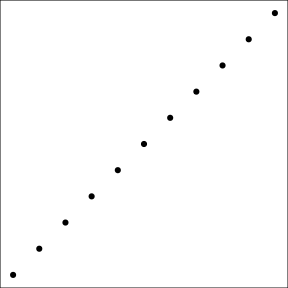Graphs from `ggplot2` can be saved, like any other R object. They can
then be reused later in other functions.

Using grobs, and `annotation_custom`:

```g1 +
annotation_custom(
grob = ggplotGrob(g1_void),
xmin = 0,
xmax = 3,
ymin = 5,
ymax = 10
) +
annotation_custom(
grob = ggplotGrob(g1_void),
xmin = 5,
xmax = 10,
ymin = 0,
ymax = 3
)```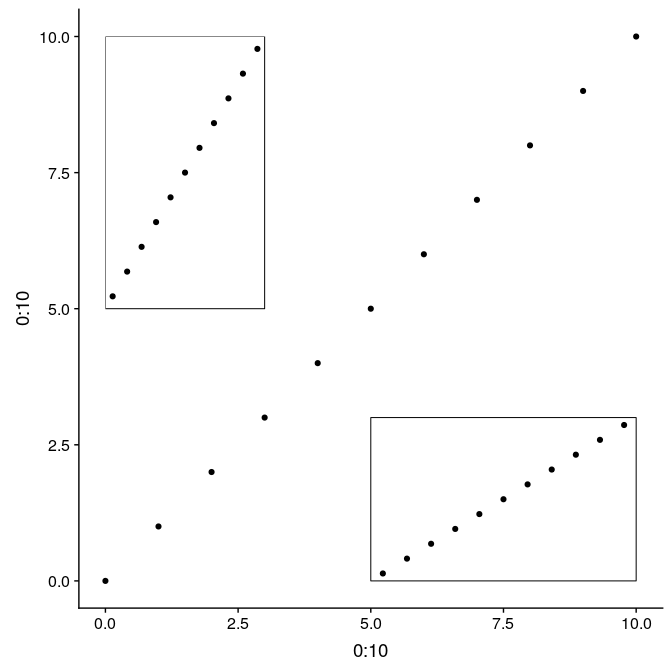Using `ggdraw` (note: used to build on top of initial plot; could be
left empty to arrange subplots on a grid; plots are “filled” with their
plots, unless the plot itself has a constrained ratio, like a map):

```ggdraw(g1) +
draw_plot(g1_void, width = 0.25, height = 0.5, x = 0.02, y = 0.48) +
draw_plot(g1_void, width = 0.5, height = 0.25, x = 0.75, y = 0.09)```# Several maps side by side or on a grid

Having a way show in a visualization, a specific area can be very
useful. Many scientists usually create maps for each specific area
individually. This is fine, but there are simpler ways to display what
is needed for a report, or publication.

This exmaple is using two maps side by side, including the legend of the
first one. It illustrates how to use a custom grid, which can be made a
lot more complex with different elements.

First, simplify `REGION` for the legend:

`levels(world\$REGION) <- "South America"`

Prepare the subplots, #1 world map:

```(gworld <- ggplot(data = world) +
geom_sf(aes(fill = REGION)) +
geom_rect(xmin = -102.15, xmax = -74.12, ymin = 7.65, ymax = 33.97,
fill = NA, colour = "black", size = 1.5) +
scale_fill_viridis_d(option = "plasma") +
theme(panel.background = element_rect(fill = "azure"),
panel.border = element_rect(fill = NA)))```And #2 Gulf map :

```(ggulf <- ggplot(data = world) +
geom_sf(aes(fill = REGION)) +
annotate(geom = "text", x = -90, y = 26, label = "Gulf of Mexico",
fontface = "italic", color = "grey22", size = 6) +
coord_sf(xlim = c(-102.15, -74.12), ylim = c(7.65, 33.97), expand = FALSE) +
scale_fill_viridis_d(option = "plasma") +
theme(legend.position = "none", axis.title.x = element_blank(),
axis.title.y = element_blank(), panel.background = element_rect(fill = "azure"),
panel.border = element_rect(fill = NA)))```The command `ggplotGrob` signals to `ggplot` to take each created map,
and how to arrange each map. The argument `coord_equal` can specify the
length, `ylim`, and width, `xlim`, for the entire plotting area. Where
as in `annotation_custom`, each maps’ `xmin`, `xmax`, `ymin`, and `ymax`
can be specified to allow for complete customization.

```#Creating a faux empty data frame
df <- data.frame()
plot1<-ggplot(df) + geom_point() + xlim(0, 10) + ylim(0, 10)
plot2<-ggplot(df) + geom_point() + xlim(0, 10) + ylim(0, 10)

ggplot() +
coord_equal(xlim = c(0, 3.3), ylim = c(0, 1), expand = FALSE) +
annotation_custom(ggplotGrob(plot1), xmin = 0, xmax = 1.5, ymin = 0,
ymax = 1) +
annotation_custom(ggplotGrob(plot2), xmin = 1.5, xmax = 3, ymin = 0,
ymax = 1) +
theme_void()```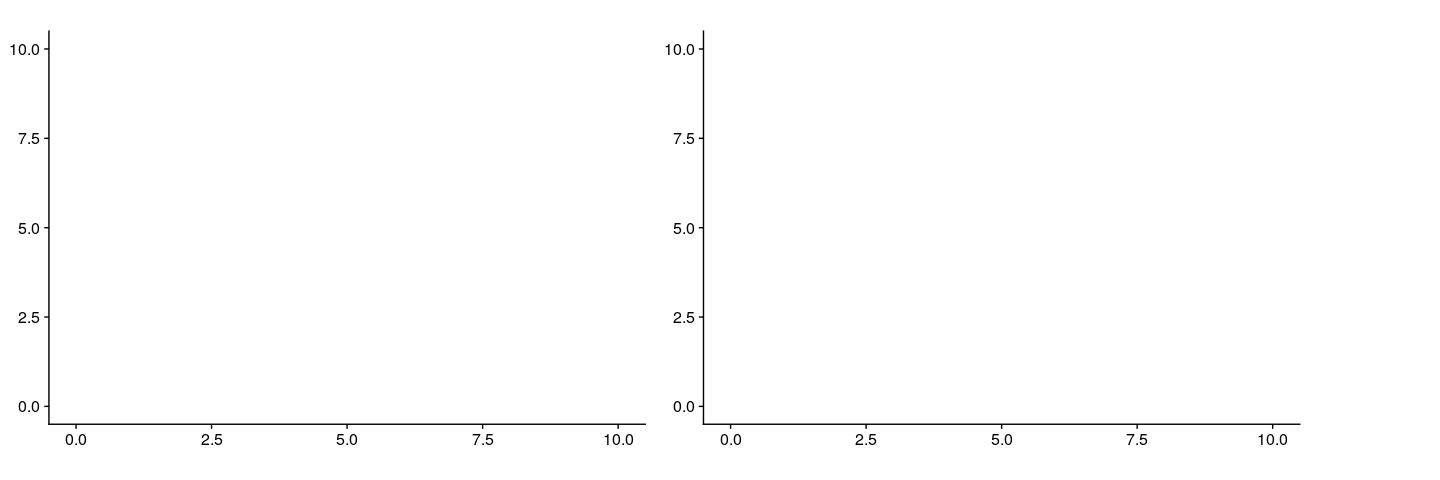Below is the final map, using the same methodology as the exmaple plot
above. Using `ggplot` to arrange maps, allows for easy and quick
plotting in one function of R code.

```ggplot() +
coord_equal(xlim = c(0, 3.3), ylim = c(0, 1), expand = FALSE) +
annotation_custom(ggplotGrob(gworld), xmin = 0, xmax = 2.3, ymin = 0,
ymax = 1) +
annotation_custom(ggplotGrob(ggulf), xmin = 2.3, xmax = 3.3, ymin = 0,
ymax = 1) +
theme_void()```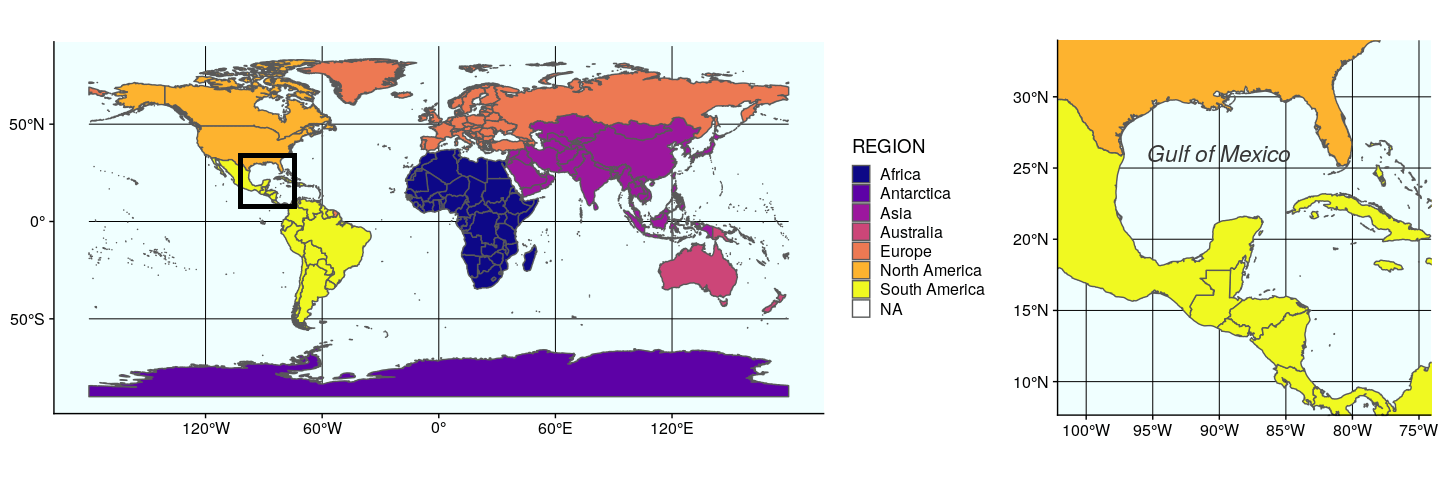In the second approach, using `cowplot::plot_grid` to arrange `ggplot`
figures, is quite versatile. Any `ggplot` figure can be arranged just
like the figure above. There are many commands that allow for the map to
have different placements, such as `nrow=1` means that the figure will
only occupy one row and multiple columns, and `ncol=1` means the figure
will be plotted on one column and multiple rows. The command
`rel_widths` establishes the width of each map, meaning that the first
map `gworld` will have a relative width of `2.3`, and the map `ggulf`
has the relative width of `1`.

```library("cowplot")
theme_set(theme_bw())

plot_grid(gworld, ggulf, nrow = 1, rel_widths = c(2.3, 1))```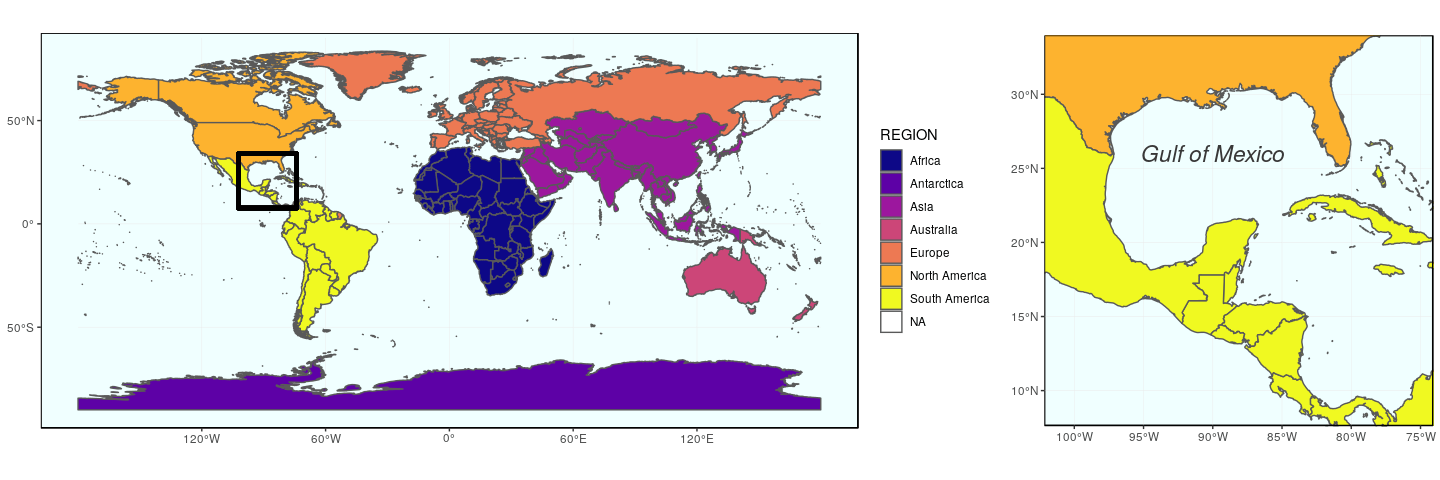Some other commands can adjust the position of the figures such as
adding `align=v` to align vertically, and `align=h` to align
horiztonally.

Note also the existence of `get_legend` (`cowplot`), and that the legend
can be used as any object.

This map can be save using,`ggsave`:

`ggsave("grid.pdf", width = 15, height =  5)`

# Map insets

For map insets directly on the background map, both solutions are viable
(and one might prefer one or the other depending on relative or absolute
coordinates).

Map example using map of the 50 states of the US, including Alaska and
Hawaii (note: not to scale for the latter), using reference projections
for US maps. First map (continental states) use a 10/6 figure:

```usa <- subset(world, ADMIN == "United States of America")
## US National Atlas Equal Area (2163)
## http://spatialreference.org/ref/epsg/us-national-atlas-equal-area/
(mainland <- ggplot(data = usa) +
geom_sf(fill = "cornsilk") +
coord_sf(crs = st_crs(2163), xlim = c(-2500000, 2500000), ylim = c(-2300000,
730000)))```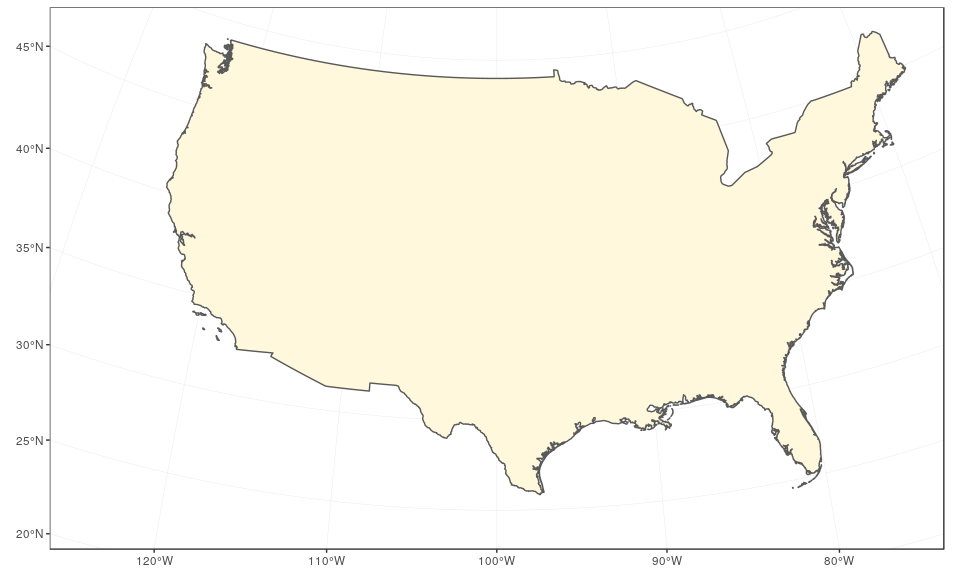Alaska map (note: `datum = NA` removes graticules and coordinates):

```## Alaska: NAD83(NSRS2007) / Alaska Albers (3467)
## http://www.spatialreference.org/ref/epsg/3467/
(alaska <- ggplot(data = usa) +
geom_sf(fill = "cornsilk") +
coord_sf(crs = st_crs(3467), xlim = c(-2400000, 1600000), ylim = c(200000,
2500000), expand = FALSE, datum = NA))```Hawaii map:

```## Hawaii: Old Hawaiian (4135)
## http://www.spatialreference.org/ref/epsg/4135/
(hawaii  <- ggplot(data = usa) +
geom_sf(fill = "cornsilk") +
coord_sf(crs = st_crs(4135), xlim = c(-161, -154), ylim = c(18,
23), expand = FALSE, datum = NA))```Using `ggdraw` from `cowplot` (tricky to define exact positions; note
the use of the ratios of the inset, combined with the ratio of the
plot):

```(ratioAlaska <- (2500000 - 200000) / (1600000 - (-2400000)))

##  0.575

(ratioHawaii  <- (23 - 18) / (-154 - (-161)))

##  0.7142857

ggdraw(mainland) +
x = 0.05, y = 0.05) +
draw_plot(hawaii, width = 0.15, height = 0.15 * 10/6 * ratioHawaii,
x = 0.3, y = 0.05)```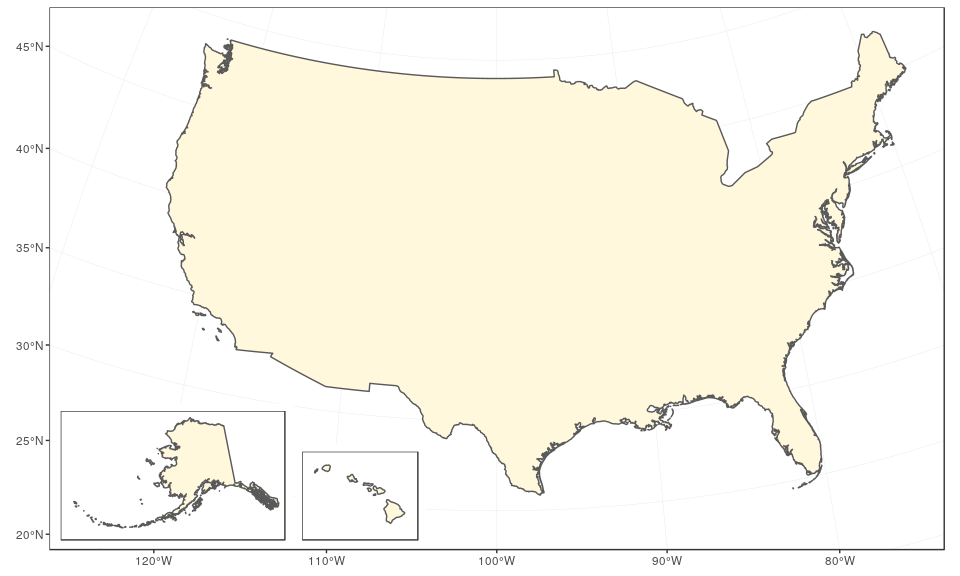This plot can be saved using `ggsave`:

`ggsave("map-us-ggdraw.pdf", width = 10, height = 6)`

The same kind of plot can be created using grobs, with `ggplotGrob`,
(note the use of xdiff/ydiff and arbitrary ratios):

```mainland +
annotation_custom(
xmin = -2750000,
xmax = -2750000 + (1600000 - (-2400000))/2.5,
ymin = -2450000,
ymax = -2450000 + (2500000 - 200000)/2.5
) +
annotation_custom(
grob = ggplotGrob(hawaii),
xmin = -1250000,
xmax = -1250000 + (-154 - (-161))*120000,
ymin = -2450000,
ymax = -2450000 + (23 - 18)*120000
)```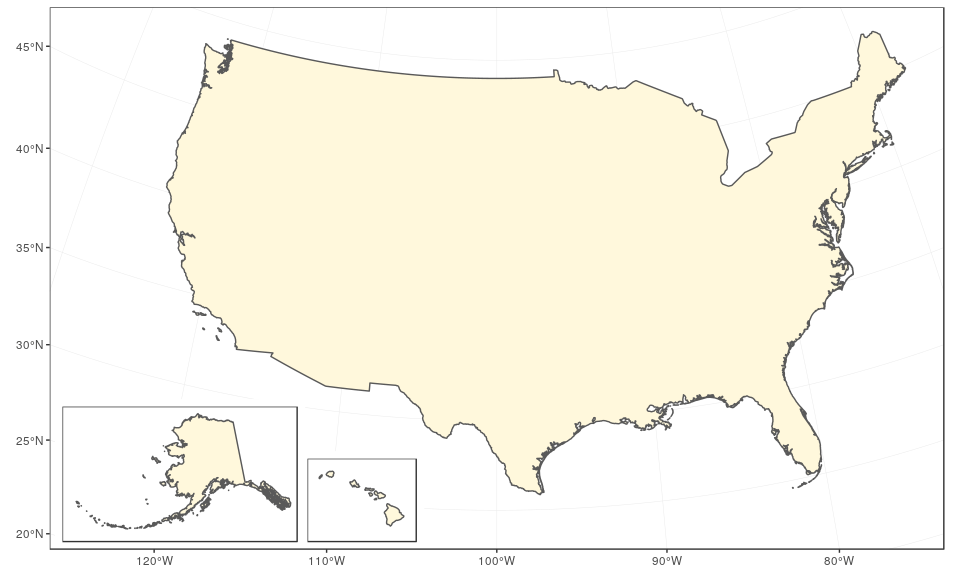This plot can be saved using `ggsave`:

`ggsave("map-inset-grobs.pdf", width = 10, height = 6)`

The `print` command can also be used place multiple maps in one plotting
area.

To specify where each plot is displayed with the `print` function, the
argument `viewport` needs to include the maximum width and height of
each map, and the minimum x and y coordinates of where the maps are
located in the plotting area. The argument `just` will make a position
on how the secondary maps will be displayed. All maps are defaulted the
same size, until the sizes are adjusted with `width` and `height`.

```vp <- viewport(width = 0.37, height = 0.10, x = 0.20, y =0.25, just = c("bottom"))
vp1<- viewport(width = 0.37, height = 0.10, x = 0.35, y =0.25, just = c("bottom"))```

The`print` function uses the previous specifications that were listed in
each plots’ respective `viewport`, with `vp=`.

```print(mainland)
print(hawaii, vp=vp1)```# Several maps connected with arrows

To bring about a more lively map arrangement, arrows can be used to
bring the viewers eyes to specific areas in the plot. The next example
will create a map with zoomed in areas, pointed to by arrows.

Firstly, we will create our main map, and then our zoomed in areas.

Site coordinates, same as Tutorial #1:

```sites <- st_as_sf(data.frame(longitude = c(-80.15, -80.1), latitude = c(26.5,
26.8)), coords = c("longitude", "latitude"), crs = 4326,
agr = "constant")```

Mainlaind map of Florida, #1:

```(florida <- ggplot(data = world) +
geom_sf(fill = "antiquewhite1") +
geom_sf(data = sites, size = 4, shape = 23, fill = "darkred") +
annotate(geom = "text", x = -85.5, y = 27.5, label = "Gulf of Mexico",
color = "grey22", size = 4.5) +
coord_sf(xlim = c(-87.35, -79.5), ylim = c(24.1, 30.8)) +
xlab("Longitude")+ ylab("Latitude")+
theme(panel.grid.major = element_line(colour = gray(0.5), linetype = "dashed",
size = 0.5), panel.background = element_rect(fill = "aliceblue"),
panel.border = element_rect(fill = NA)))```A map for site A is created by layering the map and points we created
earlier. `ggplot` layers `geom_sf` objects and plot them spatially.

```(siteA <- ggplot(data = world) +
geom_sf(fill = "antiquewhite1") +
geom_sf(data = sites, size = 4, shape = 23, fill = "darkred") +
coord_sf(xlim = c(-80.25, -79.95), ylim = c(26.65, 26.95), expand = FALSE) +
annotate("text", x = -80.18, y = 26.92, label= "Site A", size = 6) +
theme_void() +
theme(panel.grid.major = element_line(colour = gray(0.5), linetype = "dashed",
size = 0.5), panel.background = element_rect(fill = "aliceblue"),
panel.border = element_rect(fill = NA)))```A map for site B:

```(siteB <- ggplot(data = world) +
geom_sf(fill = "antiquewhite1") +
geom_sf(data = sites, size = 4, shape = 23, fill = "darkred") +
coord_sf(xlim = c(-80.3, -80), ylim = c(26.35, 26.65), expand = FALSE) +
annotate("text", x = -80.23, y = 26.62, label= "Site B", size = 6) +
theme_void() +
theme(panel.grid.major = element_line(colour = gray(0.5), linetype = "dashed",
size = 0.5), panel.background = element_rect(fill = "aliceblue"),
panel.border = element_rect(fill = NA)))```Coordinates of the two arrows will need to be specified before plotting.
The argumemnts `x1`, and `x2` will plot the arrow line from a specific
starting x-axis location,`x1`, and ending in a specific x-axis,`x2`. The
same applies for `y1` and `y2`, with the y-axis respectively:

```arrowA <- data.frame(x1 = 18.5, x2 = 23, y1 = 9.5, y2 = 14.5)
arrowB <- data.frame(x1 = 18.5, x2 = 23, y1 = 8.5, y2 = 6.5)```

Final map using (`ggplot` only). The argument `geom_segment`, will be
the coordinates created in the previous script, to plot line segments
ending with an arrow using `arrow=arrow()`:

```ggplot() +
coord_equal(xlim = c(0, 28), ylim = c(0, 20), expand = FALSE) +
annotation_custom(ggplotGrob(florida), xmin = 0, xmax = 20, ymin = 0,
ymax = 20) +
annotation_custom(ggplotGrob(siteA), xmin = 20, xmax = 28, ymin = 11.25,
ymax = 19) +
annotation_custom(ggplotGrob(siteB), xmin = 20, xmax = 28, ymin = 2.5,
ymax = 10.25) +
geom_segment(aes(x = x1, y = y1, xend = x2, yend = y2), data = arrowA,
arrow = arrow(), lineend = "round") +
geom_segment(aes(x = x1, y = y1, xend = x2, yend = y2), data = arrowB,
arrow = arrow(), lineend = "round") +
theme_void()```This plot can be saved using `ggsave`:

`ggsave("florida-sites.pdf", width = 10, height = 7)`

`ggdraw` could also be used for a similar result, with the argument
`draw_plot`:

```ggdraw(xlim = c(0, 28), ylim = c(0, 20)) +
draw_plot(florida, x = 0, y = 0, width = 20, height = 20) +
draw_plot(siteA, x = 20, y = 11.25, width = 8, height = 8) +
draw_plot(siteB, x = 20, y = 2.5, width = 8, height = 8) +
geom_segment(aes(x = x1, y = y1, xend = x2, yend = y2), data = arrowA,
arrow = arrow(), lineend = "round") +
geom_segment(aes(x = x1, y = y1, xend = x2, yend = y2), data = arrowB,
arrow = arrow(), lineend = "round")```